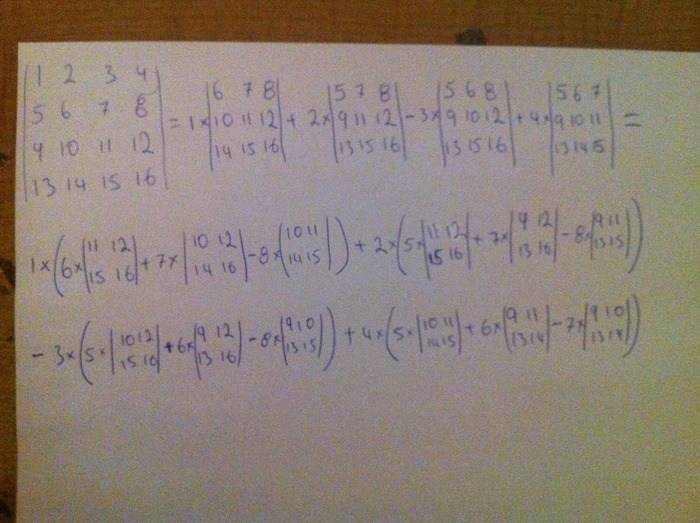# How to find the value of λ inside a 4x4 matrix that results in determinant = 0

## Homework Statement

As you can see from the picture below this a 4x4 matrix with an unknown value, λ. My objective here is to find four values for λ so that the determinant of the 4x4 matrix is equal to zero.

http://i49.tinypic.com/n4cenn.png

## Homework Equations

The above 4x4 matrix is the result of this equation

|[K]- λ[M]|

Where:

http://i50.tinypic.com/fdrgy1.jpg

## The Attempt at a Solution

I have tried to use excel to solve this through iteration but couldnt make it how ever i did try different values for λ but the answer that i get for the determinant is always too high.

i think there are 3 possible ways to solve this: either by hand calculations which i do not know how to do or by using excel to program a function for iteration or again by using excel and plotting two columns => 1st column (values of λ inside the matrix) which will change automatically giving the result of the determinant in the 2nd column from which a graph can be plotted and the values of λ could be read from the graph which i also tried to do but i was unable to make the values of λ inside the matrix change automatically and follow the 1st column which contained all the values for λ.

Last edited by a moderator:

## Answers and Replies

Mute
Homework Helper
If you want to learn how to do this by hand, read up on the Laplace expansion. Particularly, see the example. It works similarly for 4x4 as for the 3x3 matrix in the article. We can help you do the calculation here. You will get a quartic polynomial for ##\lambda## which you can then solve numerically (using excell or wolframalpha or something).

Since the matrix is 4x4 the expansion is not too hard to do, but you will probably not want to do it by hand for larger matrices.

I'm afraid I don't really know how to solve the determinant numerically in Excell. For programs like C, a library such as the Gnu Scientific Library (GSL) will have code for calculating the eigenvalues of large (though usually sparce) matrices. Off the top of my head I don't know if they can do your more generalized case of ##\mbox{det}(K-\lambda M) = 0##, though if M has an inverse your problem is equivalent to ##\mbox{det}(KM^{-1}-\lambda I) = 0##.

Edit: Actually, the GSL does have solvers for generalized eigensystems, ##K\mathbf{v} = \lambda M \mathbf{v}##. You need to know C to use this though.

Last edited:
Hey thanks a lot for your reply. I think i will try to solve this by hand and then solve the quartic polynomial in wolframalpha as you suggested, however since i have not tried to solve a 4x4 matrix before i will post a picture of an example of how i think this can be solved so that you can let me know if i got it right in my mind.

One more question, lets say i have solved the 4x4 matrix and end up with the quatric polynomial equation how can i be 100% sure that there are four values of λ that will make the whole equation = 0

epenguin
Homework Helper
Gold Member
One more question, lets say i have solved the 4x4 matrix and end up with the quatric polynomial equation how can i be 100% sure that there are four values of λ that will make the whole equation = 0[/QUOTE]

You can be 100% sure of that last - it's called the fundamental theorem of algebra - that an n-degree polynomial equation has n roots (possibly some coincident).
If the question is how to be sure whether they are all real (two or four of them might be nonreal complex numbers) and that they are not double roots that is more complicatedbut I think that computer program gives you those roots too.

Hi, i did not got back to my post for a while because we managed to solve this and found all four values for λ by using iteration in excel. However the determinant was not exactly zero with the results we got but we were allowed to use them. Moreover i was interested to see how a 4x4 matrix is solved and i have posted a picture below showing an example of how i think it can be solved. Please let me know if this is the correct way.

In the picture below the process is not completed however is my approach correct?STEMucator
Homework Helper
Looks good.

ok thanks alot